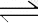Equilibrium Le Chatelier's Principle Volume change of an aqueous mixture. According to Le Chatelier's Principle diluting the equilibrium mixture by increasing the volume would cause the system to move in the direction of increasing the number of particles and therefore increasing the concentration. Reducing the volume and therefore increasing the concentration will have the effect of driving the reaction towards the side with least particles. For example, take the reaction represented by the equation below. Fe3+(aq) + SCN- (aq)FeSCN2+(aq) Increasing the volume of the equilibrium mixture drives this reaction to the left. A decrease in volume would drive the forward reaction. Similarly the reaction shown on the right, would be driven to the left if the equilibrium mixture was diluted. This reaction gives a striking colour change as it moves to the left.   Co2+(aq) + 4Cl-(aq)CoCl4-2(aq) Continue